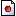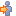# LlVecNorm

(diff) ← Older revision | Latest revision (diff) | Newer revision → (diff)

 LSL Portal
Function: vector llVecNorm( vector vec );
 13 Function ID 0 Forced Delay 10 Energy

Returns the vector that is vec normalized (a unit vector sharing the same direction as vec).

 • vector vec – Any valid vector

If vec is a ZERO_VECTOR, than the value returned is a ZERO_VECTOR.

## Caveats

#### Important IssuesSVC-4711 llVecNorm returns zero vector as result when input is below a certain magnitude

## Examples

```default {
state_entry()
{
vector input = <1.0,2.0,3.0>;
llSay(0,"The unit vector on "+(string)input+" is: "+(string)llVecNorm(input) );
}
}
```

### Functions

 • llVecMag • llVecDist

## Deep Notes

#### All IssuesSVC-4711 llVecNorm returns zero vector as result when input is below a certain magnitude

#### Signature

```function vector llVecNorm( vector vec );
```